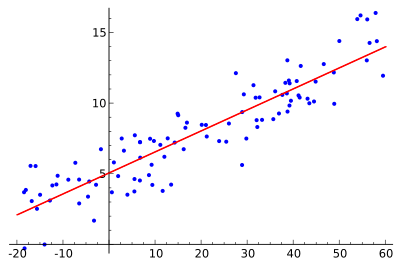Thanks to theidioms.com### Infosys Tech Scholars Scholarship## R lm() – lm function in R for Linear Regression# R lm() – lm function in R for Linear Regression

Greetings! Some links on this site are affiliate links. That means that, if you choose to make a purchase, The Click Reader may earn a small commission at no extra cost to you. We greatly appreciate your support!

The R lm() function is used to fit linear models for performing linear regression, single stratum analysis of variance, and analysis of covariance.

The usage of the lm() function in R is as follows:

```lm(formula, data, subset, weights, na.action,
method = "qr", model = TRUE, x = FALSE, y = FALSE, qr = TRUE,
singular.ok = TRUE, contrasts = NULL, offset, …)```

Here, the parameters/arguments are defined as:

• formula: an object of class `"formula"` (or one that can be coerced into that class): a symbolic description of the model to be fitted.
• data: an optional data frame, list or environment (or object coercible by `as.data.frame` to a data frame) containing the variables in the model. If not found in `data`, the variables are taken from `environment(formula)`, typically the environment from which `lm` is called.
• subset: an optional vector specifying a subset of observations to be used in the fitting process.
• weights: an optional vector of weights to be used in the fitting process. Should be `NULL` or a numeric vector. If non-NULL, weighted least squares is used with weights `weights` (that is, minimizing `sum(w*e^2)`); otherwise ordinary least squares is used.
• na.action: a function which indicates what should happen when the data contain `NA`s. The default is set by the `na.action` setting of `options`, and is `na.fail` if that is unset. The ‘factory-fresh’ default is `na.omit`. Another possible value is `NULL`, no action. Value `na.exclude` can be useful.
• method: the method to be used; for fitting, currently only `method = "qr"` is supported; `method = "model.frame"` returns the model frame (the same as with `model = TRUE`).
• model, x, y, q: If `TRUE` the corresponding components of the fit (the model frame, the model matrix, the response, the QR decomposition) are returned.
• singular.ok: If `FALSE` (the default in S but not in R) a singular fit is an error.
• contrasts: an optional list.
• offset: this can be used to specify an a priori known component to be included in the linear predictor during fitting. This should be `NULL` or a numeric vector or matrix of extents matching those of the response. One or more `offset` terms can be included in the formula instead or as well, and if more than one are specified their sum is used.
• …: additional arguments to be passed to the low level regression fitting functions.

## Example Implementation of R lm() function:

The lm() function can be implemented in R according to the following example:

```library(readxl) # Library for reading excel files
lmHeight = lm(height~age, data = ageandheight) # Create linear regression model using lm
summary(lmHeight) # Review the results ```

In the example above, you can substitute `ageandheight.xls` to be any dataset that you want.Do you want to learn Python, Data Science, and Machine Learning while getting certified? Here are some best selling Datacamp courses that we recommend you enroll in:

1. Introduction to Python (Free Course) - 1,000,000+ students already enrolled!
2. Introduction to Data Science  in Python- 400,000+ students already enrolled!
3. Introduction to TensorFlow for Deep Learning with Python - 90,000+ students already enrolled!
4. Data Science and Machine Learning Bootcamp with R - 70,000+ students already enrolled!